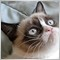How to remove zeros from array?965

for(int x=1;x<50;x++) // GET CLOSES THAT ARE LOWER THAN PREVIOUS CANDLE'S CLOSE
if(iClose(_Symbol,0,x)<iClose(_Symbol,0,x+1))
ClosesBelowPreviousArray[x]=x;
// NOW WE ATTEMPT TO REMOVE ZEROS:
for(int x=1;x<50;x++)
if(ClosesBelowPreviousArray[x]>0)
{
Print("ClosesBelowPreviousArray[",x,"] ",ClosesBelowPreviousArray[x]); // HOW TO GET AN ARRAY THAT JUST HAS AN ORDERED FROM ARRAY TO ARRAY AN ASCENDING ORDERED LIST OF NUMBERS WITHOUT ZEROS JUST LIKE THIS PRINT COMMAND DISPLAYS BUT IN A FROM ARRAY to ARRAY ARRAY?
}

Ive tried

for(int x=1;x<50;x++) // GET CLOSES THAT ARE LOWER THAN PREVIOUS CANDLE'S CLOSE
if(iClose(_Symbol,0,x)<iClose(_Symbol,0,x+1))
ClosesBelowPreviousArray[x]=x;
// NOW WE ATTEMPT TO REMOVE ZEROS:
for(int x=1;x<50;x++)
if(ClosesBelowPreviousArray[x]>0)
{
for(int y=1;y<ArraySize(ClosesBelowPreviousArrayNoZeros);y++) // ORDER THEM WITHOUT ZEROS FOR SIMPLY AN ASCENDING FROM 1 TO ARRAY SIZE ORDERED LIST WITH A NESTED LOOP POSSIBLE?
ClosesBelowPreviousArrayNoZeros[y]=x;
}

for(int x=1;x<ArraySize(ClosesBelowPreviousArrayNoZeros);x++)
Print("ClosesBelowPreviousArrayNoZeros[",x,"] ",ClosesBelowPreviousArray[x]); // same as normal array , stil has zeros!! any ideas?

Cant find a way to remove zeros from the ClosesBelowPreviousArray array. We need to to keep the actual order in which the candle's close was less than the previous candle's close. So the result we need is

ClosesBelowPreviousArrayNoZeros // the shift where the candle's close was first  less than previous

ClosesBelowPreviousArrayNoZeros // the second time where shift where the candle's close was less than previous

ClosesBelowPreviousArrayNoZeros // the third occurence when shift where the candle's close was less than previous

ClosesBelowPreviousArrayNoZeros // the shit of fourth time when the candle's close was less than previous

Any way to order the ClosesBelowPreviousArray[] by removing the zeros? The result would be the Print of the first code however in array form with the indexes from 1 to 10 instead of where the condition happen followed by a ton of zeros and then again another x="where the condition happens" again.

Just making all the zeros into 999999 and then get the ArrayMin, assign this to a clunky sounding variable and then deleting the array min from array and repeating this forever made me post this.862

int count=0;
double closesNoZeroes;

for(int x=1;x<50;x++) // GET CLOSES THAT ARE LOWER THAN PREVIOUS CANDLE'S CLOSE
if(iClose(_Symbol,0,x)<iClose(_Symbol,0,x+1))
{
ClosesBelowPreviousArray[x]=x;
closesNoZeroes[count++]=x;
}
ArrayResize(closesNoZeroes,count); // shrink to true size
This is a quick way to do the job. Note that closesNoZeroes is a dynamic array and so maybe resized to fit its contents, that's what is done at the end.965

lippmaje:
This is a quick way to do the job. Note that closesNoZeroes is a dynamic array and so maybe resized to fit its contents, that's what is done at the end.

THANK YOU SO MUCH!!

closesNoZeroes[count++]

GENIUS862

👍